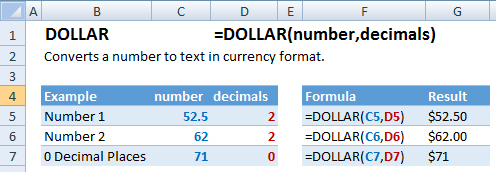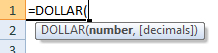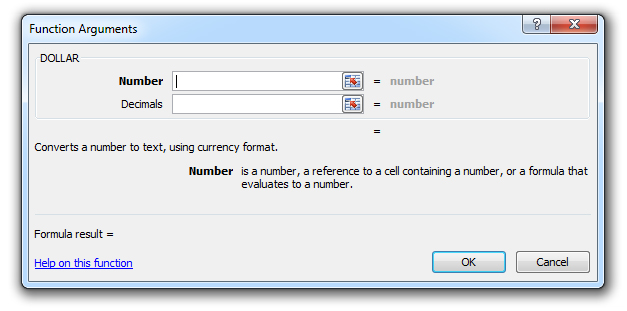### DOLLAR Function – Dollar Sign – Excel, VBA, & Google Sheets

This Excel Tutorial demonstrates how to use the Excel DOLLAR Function in Excel to convert a number to text and add a dollar sign, with formula examples.

## DOLLAR Function Description:

The DOLLAR Function Converts a number to text and stores the text in currency format.

## Formula Examples:Example Formula Result
Number 1 =DOLLAR(C5,D5) \$52.50
Number 2 =DOLLAR(C6,D6) \$62.00
0 Decimal Places =DOLLAR(C7,D7) \$71
AutoMacro - VBA Code Generator

## Syntax and Arguments:

The Syntax for the DOLLAR Formula is:

`=DOLLAR(number,decimals)`

### Function Arguments ( Inputs ):

number – The number you wish to convert into text formatted as currency.

decimals – OPTIONAL. The number of decimal places to display. Default = 2.

## Additional Notes

Using the DOLLAR Function will convert the number into a string of text in dollar format. To simply convert the format of the cell, while keeping the number stored as a number, use the Format Cells Dialog Box ( Shortcut: CTRL + 1 ) or make the change in the Home Ribbon. <>.

## DOLLAR Examples in VBA

You can also use the DOLLAR function in VBA. Type:
`application.worksheetfunction.dollar(number,decimals)`
For the function arguments (number, etc.), you can either enter them directly into the function, or define variables to use instead.

Return to the List of all Functions in Excel

## How to use the DOLLAR Function in Excel:

To use the AND Excel Worksheet Function, type the following into a cell:
`=AND(`
After entering it in the cell, notice how the AND formula inputs appear below the cell:You will need to enter these inputs into the function. The function inputs are covered in more detail in the next section. However, if you ever need more help with the function, after typing “=DOLLAR(” into a cell, without leaving the cell, use the shortcut CTRL + A (A for Arguments) to open the “Insert Function Dialog Box” for detailed instructions:For more information about the DOLLAR Formula visit the
Microsoft Website.

## Excel Practice Worksheet

Practice Excel functions and formulas with our 100% free practice worksheets!

• Automatically Graded Exercises
• Learn Excel, Inside Excel!